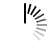# Fortran Programming Examples

## Hello World Program in FORTRAN-95

```program hello
Print *, "Hello World!...4...."
end program Hello```

## Printing Statement multiple times using Fortran

```program name
!this program prints my name to the screen
integer::i
do i=1,10
print*,'my name is raksha'
end do
end program name```

## t26

```program t26
! max value of three numbers
integer::x,y,z
read*,x,y,z
print*,"x=",x
print*,"y=",y
print*,'z=',z
if (x>y) then
if (y>a)then
max=x
else
max=z
end if
end if
if(y>z)then
max=y
end if
print*,'max value of three numbers is',max
end program t26```

## t26

```program t26
! max value of three numbers
integer:: a,b,c
read*, a,b,c
print*,"a=",a
print*,"b=",b
print*,"c=",c
if(a>b)then
if(a>c)then
max=a
else
max=c
end if
end if
if(b>c)then
max=b
else
max=c
end if
print*,"max value of three number is",max
end program t26```

## sum25

```program sum25
! count and print all the numbers from low to high
integer::low,high,step,a
read*,low,high,step
do a=low,high,step
print*,a
end do
end program sum25```

## T25

```program T25
!count and print all numbers from low to high
integer::low,high,step,a
read*,low,high,step
do a=low,high,step
print*,a
end do
end program T25```

## Prob 5

```program ex5
!Write an algorithm to count and print all numbers from LOW to HIGH
integer::low,high,step,c
read*,low,high,step
do c=low,high,step
print*,c
end do
end program ex5```

## Compile and Execute FORTRAN-95 Online

```program maxnumber
integer::i, a(100)
read *,n
do i=1,n
read*, a(i)
print *, 'a(',i,')=',a(i)
max=-9999999
if (a(i)>max) then
max=a(i)
end if
end do
print *, max

end program maxnumber```

## t25

```program t25
! print all numbers from low to high
integer:: low,high,step
do a=low,high,step
print*,a
end do
end program t25```

## tprob2

```program prob2
!double sum of number frm low to high
integer :: i,s,low,high
read *, low,high
print *, 'low' ,low, 'high' ,high
s=0
do i=low,high
s=2*(i+s)
print *, 'double sum' ,s
end do
end program prob2```

AdvertisementsWe use cookies to provide and improve our services. By using our site, you consent to our Cookies Policy.